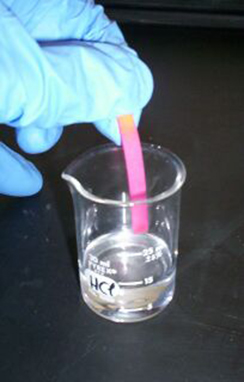# 6.5 Logarithmic properties

 Page 1 / 10
In this section, you will:
• Use the product rule for logarithms.
• Use the quotient rule for logarithms.
• Use the power rule for logarithms.
• Expand logarithmic expressions.
• Condense logarithmic expressions.
• Use the change-of-base formula for logarithms.The pH of hydrochloric acid is tested with litmus paper. (credit: David Berardan)

In chemistry, pH is used as a measure of the acidity or alkalinity of a substance. The pH scale runs from 0 to 14. Substances with a pH less than 7 are considered acidic, and substances with a pH greater than 7 are said to be alkaline. Our bodies, for instance, must maintain a pH close to 7.35 in order for enzymes to work properly. To get a feel for what is acidic and what is alkaline, consider the following pH levels of some common substances:

• Battery acid: 0.8
• Stomach acid: 2.7
• Orange juice: 3.3
• Pure water: 7 (at 25° C)
• Human blood: 7.35
• Fresh coconut: 7.8
• Sodium hydroxide (lye): 14

To determine whether a solution is acidic or alkaline, we find its pH, which is a measure of the number of active positive hydrogen ions in the solution. The pH is defined by the following formula, where $\text{\hspace{0.17em}}a\text{\hspace{0.17em}}$ is the concentration of hydrogen ion in the solution

The equivalence of $\text{\hspace{0.17em}}-\mathrm{log}\left(\left[{H}^{+}\right]\right)\text{\hspace{0.17em}}$ and $\text{\hspace{0.17em}}\mathrm{log}\left(\frac{1}{\left[{H}^{+}\right]}\right)\text{\hspace{0.17em}}$ is one of the logarithm properties we will examine in this section.

## Using the product rule for logarithms

Recall that the logarithmic and exponential functions “undo” each other. This means that logarithms have similar properties to exponents. Some important properties of logarithms are given here. First, the following properties are easy to prove.

$\begin{array}{l}{\mathrm{log}}_{b}1=0\\ {\mathrm{log}}_{b}b=1\end{array}$

For example, $\text{\hspace{0.17em}}{\mathrm{log}}_{5}1=0\text{\hspace{0.17em}}$ since $\text{\hspace{0.17em}}{5}^{0}=1.\text{\hspace{0.17em}}$ And $\text{\hspace{0.17em}}{\mathrm{log}}_{5}5=1\text{\hspace{0.17em}}$ since $\text{\hspace{0.17em}}{5}^{1}=5.$

Next, we have the inverse property.

For example, to evaluate $\text{\hspace{0.17em}}\mathrm{log}\left(100\right),$ we can rewrite the logarithm as $\text{\hspace{0.17em}}{\mathrm{log}}_{10}\left({10}^{2}\right),$ and then apply the inverse property $\text{\hspace{0.17em}}{\mathrm{log}}_{b}\left({b}^{x}\right)=x\text{\hspace{0.17em}}$ to get $\text{\hspace{0.17em}}{\mathrm{log}}_{10}\left({10}^{2}\right)=2.$

To evaluate $\text{\hspace{0.17em}}{e}^{\mathrm{ln}\left(7\right)},$ we can rewrite the logarithm as $\text{\hspace{0.17em}}{e}^{{\mathrm{log}}_{e}7},$ and then apply the inverse property $\text{\hspace{0.17em}}{b}^{{\mathrm{log}}_{b}x}=x\text{\hspace{0.17em}}$ to get $\text{\hspace{0.17em}}{e}^{{\mathrm{log}}_{e}7}=7.$

Finally, we have the one-to-one property.

We can use the one-to-one property to solve the equation $\text{\hspace{0.17em}}{\mathrm{log}}_{3}\left(3x\right)={\mathrm{log}}_{3}\left(2x+5\right)\text{\hspace{0.17em}}$ for $\text{\hspace{0.17em}}x.\text{\hspace{0.17em}}$ Since the bases are the same, we can apply the one-to-one property by setting the arguments equal and solving for $\text{\hspace{0.17em}}x:$

But what about the equation $\text{\hspace{0.17em}}{\mathrm{log}}_{3}\left(3x\right)+{\mathrm{log}}_{3}\left(2x+5\right)=2?\text{\hspace{0.17em}}$ The one-to-one property does not help us in this instance. Before we can solve an equation like this, we need a method for combining terms on the left side of the equation.

Recall that we use the product rule of exponents to combine the product of exponents by adding: $\text{\hspace{0.17em}}{x}^{a}{x}^{b}={x}^{a+b}.\text{\hspace{0.17em}}$ We have a similar property for logarithms, called the product rule for logarithms , which says that the logarithm of a product is equal to a sum of logarithms. Because logs are exponents, and we multiply like bases, we can add the exponents. We will use the inverse property to derive the product rule below.

Given any real number $\text{\hspace{0.17em}}x\text{\hspace{0.17em}}$ and positive real numbers and $\text{\hspace{0.17em}}b,$ where $\text{\hspace{0.17em}}b\ne 1,$ we will show

#### Questions & Answers

find general solution of the Tanx=-1/root3,secx=2/root3
find general solution of the following equation
Nani
the value of 2 sin square 60 Cos 60
0.75
Lynne
0.75
Inkoom
when can I use sin, cos tan in a giving question
depending on the question
Nicholas
I am a carpenter and I have to cut and assemble a conventional roof line for a new home. The dimensions are: width 30'6" length 40'6". I want a 6 and 12 pitch. The roof is a full hip construction. Give me the L,W and height of rafters for the hip, hip jacks also the length of common jacks.
John
I want to learn the calculations
where can I get indices
I need matrices
Nasasira
hi
Raihany
Hi
Solomon
need help
Raihany
maybe provide us videos
Nasasira
Raihany
Hello
Cromwell
a
Amie
What do you mean by a
Cromwell
nothing. I accidentally press it
Amie
you guys know any app with matrices?
Khay
Ok
Cromwell
Solve the x? x=18+(24-3)=72
x-39=72 x=111
Suraj
Solve the formula for the indicated variable P=b+4a+2c, for b
Need help with this question please
b=-4ac-2c+P
Denisse
b=p-4a-2c
Suddhen
b= p - 4a - 2c
Snr
p=2(2a+C)+b
Suraj
b=p-2(2a+c)
Tapiwa
P=4a+b+2C
COLEMAN
b=P-4a-2c
COLEMAN
like Deadra, show me the step by step order of operation to alive for b
John
A laser rangefinder is locked on a comet approaching Earth. The distance g(x), in kilometers, of the comet after x days, for x in the interval 0 to 30 days, is given by g(x)=250,000csc(π30x). Graph g(x) on the interval [0, 35]. Evaluate g(5)  and interpret the information. What is the minimum distance between the comet and Earth? When does this occur? To which constant in the equation does this correspond? Find and discuss the meaning of any vertical asymptotes.
The sequence is {1,-1,1-1.....} has
circular region of radious
how can we solve this problem
Sin(A+B) = sinBcosA+cosBsinA
Prove it
Eseka
Eseka
hi
Joel
yah
immy
June needs 45 gallons of punch. 2 different coolers. Bigger cooler is 5 times as large as smaller cooler. How many gallons in each cooler?
7.5 and 37.5
Nando
how would this look as an equation?
Hayden
5x+x=45
Khay
find the sum of 28th term of the AP 3+10+17+---------
I think you should say "28 terms" instead of "28th term"
Vedant
the 28th term is 175
Nando
192
Kenneth
if sequence sn is a such that sn>0 for all n and lim sn=0than prove that lim (s1 s2............ sn) ke hole power n =nByBy Subramanian DivyaByBy